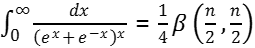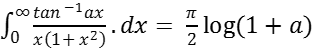MORE IN Applied Mathematics 2
MU First Year Engineering (Semester 2)
Applied Mathematics 2
May 2012
Total marks: --
Total time: --
INSTRUCTIONS
(1) Assume appropriate data and state your reasons
(2) Marks are given to the right of every question
(3) Draw neat diagrams wherever necessary

1(a) Evaluate the following:5 M
1(b) Solve the following:5 M
1(c) Solve the following:5 M
1(d) Find by double integration the area enclosed by y2 = x3, y = x.
5 M

2(a) Solve (4xy + 3y2 - x) dx +x(x+2y)dy
6 M
2(b) Change the order of integration:6 M
2(c) Prove that:Hence evaluate:8 M

3(a) Using Euler's method find approximate value of y at x=1 in five steps taking h=0.2 given dy/dx = x + y, and y(0) = 1.
6 M
3(b) Evaluate the following6 M
3(c) Solve the following:8 M

4(a) Show that the following holds true: :6 M
4(b) Evaluate the following, where R is the region bounded by y2=ax and y = x.
$\displaystyle\int\limits_R\displaystyle\int \dfrac{y\ dx\ dy}{(a-x)\sqrt{ax-y^2}}$
6 M
4(c) Solve by method of variation of parameters (D2 - 2D + 2)y = extan(x)
8 M

5(a) Solve the following:  (D2 + 2)y = excos(x) + x2e3x

6 M
5(b) Using Taylor's Method solve the following: dy/dx = x2 - y with y(0) = 1. Also find y at x - 0.1.
6 M
5(c) Find the Volume of the Tetrahedron bounded by the planes: x = 0, y = 0, z = 0 and x + y + z = a
8 M

6(a) In a single closed circuit, the current i at any time t, is given by: R i + L (di/dt) = E.
Find the current i at a time t if at t=0, i=0 and L, R, E are constants.
6 M
6(b) Find the mass of the octant of the ellipsoid x2/a2 + y2/b2 + z2/c2 =1

, the density at any point being kxyz.
6 M
6(c) Using Runge-Kutta's Fourth order method find y at x = 0.2 if dy/dx = x + y2 given that y = 1, when x = 0 in steps of h = 0.1.
8 M

7(a) State and prove Duplication formula for gamma functions.
6 M
7(b) Find the length of the cardiode r = a(1 + cosθ) which lies outside the circle r + acosθ = 0
6 M
7(c) Solve the following:8 M

More question papers from Applied Mathematics 2